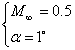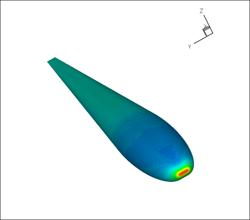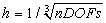Problem C2.3. Analytical 3D Body of Revolution

Overview

This problem is aimed at testing high-order methods for the computation of external flow with a high-order curved boundary representation in 3D. Inviscid, viscous (laminar) and turbulent flow conditions will be simulated.

Governing Equations

The governing equations for inviscid and laminar flows are the 3D Euler and Navier-Stokes equations with a constant ratio of specific heats of 1.4 and Prandtl number of 0.72. For the laminar flow problem, the viscosity is assumed a constant.

Flow Conditions

Inviscid:Laminar:Turbulent:Geometry

The geometry is a streamlined body based on a 10 percent thick airfoil with boundaries constructed by a surface of revolution. The airfoil is constructed by an elliptical leading edge and straight lines.

Half model:Figure 3D Body of Revolution

Reference values

Reference area: 0.1 (full model)

Reference moment length: 1.0

Moment line: Quarter chord

Boundary Conditions

Far field boundary: Subsonic inflow and outflow

Wing surface: no slip adiabatic wall

Requirements

1.     Start the simulation from a uniform free stream everywhere, and monitor the L2 norm of the density residual. Track the work units needed to achieve steady state. Compute the drag and lift coefficients cd and cl.

2.     Perform grid and order refinement studies to find “converged” cd and cl values.

3.     Plot the cd and cl errors vs. work units.

4.     Study the numerical order of accuracy according to cd and cl errors vs..

5.     Submit two sets of data to the workshop contact for this case

a)     cd and cl error vs. work units

b)     cd and cl error vs